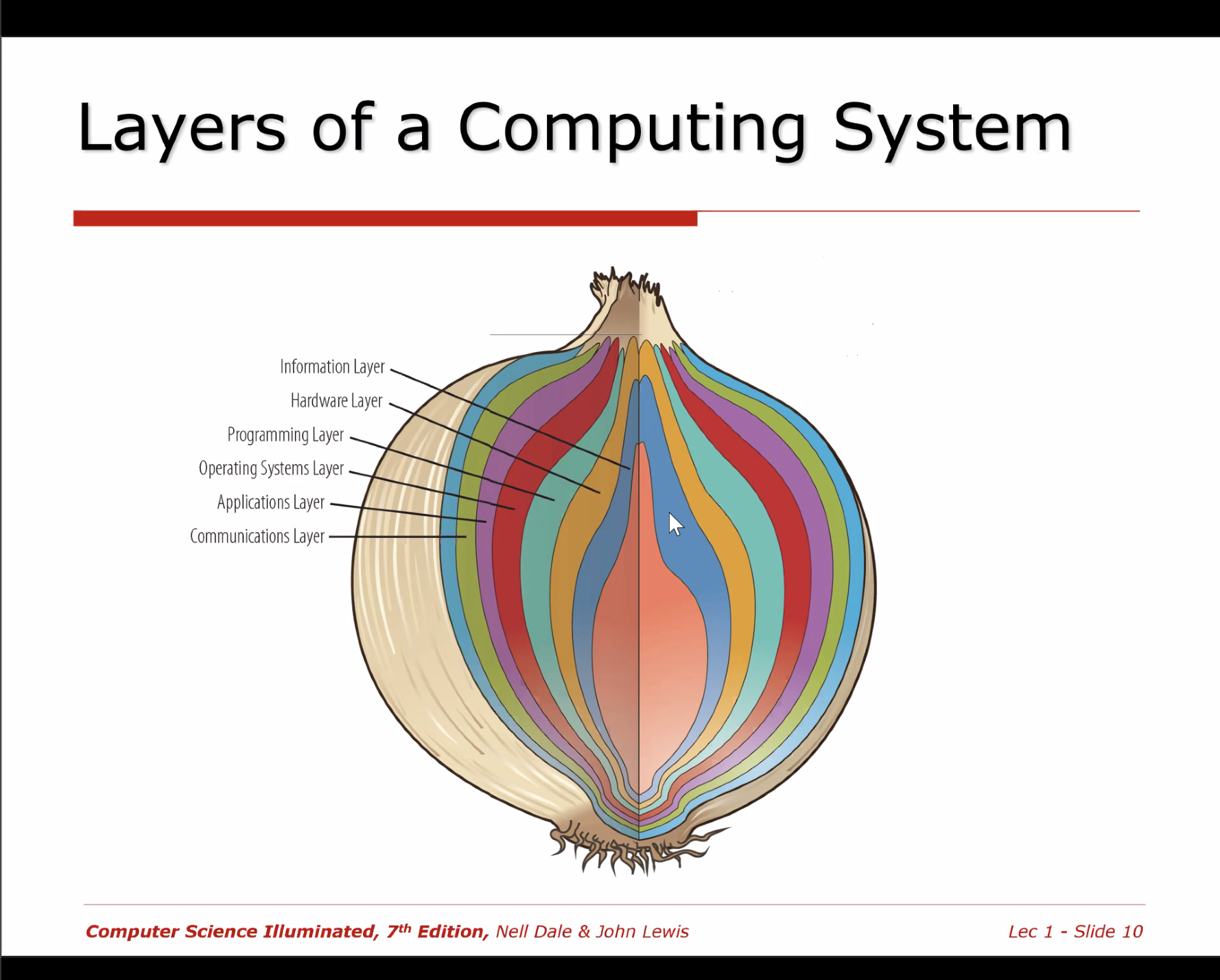🧠

# Computing Systems

• Dynamic entity used to solve problems
• Composed of hardware, software, and data
• Hardware: physical elements of computing system
• Software: Programs providing instructions for system to execute

## Layers• Information Layer
• The way we represent information
• Mostly conceptual
• Hardware Layer
• Programming Layer
• Software
• Operating Systems Layer
• Help us interact with the computer system
• Manage the way hardware, programs, and data interact
• Applications Layer
• Using the computer to solve real world problems
• Communications Layer

## Prefixes

Power of 10 Power of 2 Value of Power of 2 Prefix Abbreviation Derivation
$10^{-12}$ pico- p Italian for little
$10^{-9}$ nano- n Greek for dwarf
$10^{-6}$ micro- μ Greek for small
$10^{-3}$ milli- m Latin for thousandth
$10^{3}$ 2^{10} 1024 kilo- K Greek for thousand
$10^{6}$ 2^{20} 1,048,576 mega- M Greek for large
$10^{9}$ 2^{30} 1,073,741,824 giga- G Greek for giant
$10^{12}$ 2^{40} not enough room tera- T Greek for monster
$10^{15}$ 2^{50} not enough room peta- P Greek for five

Computer Science